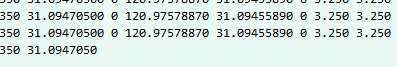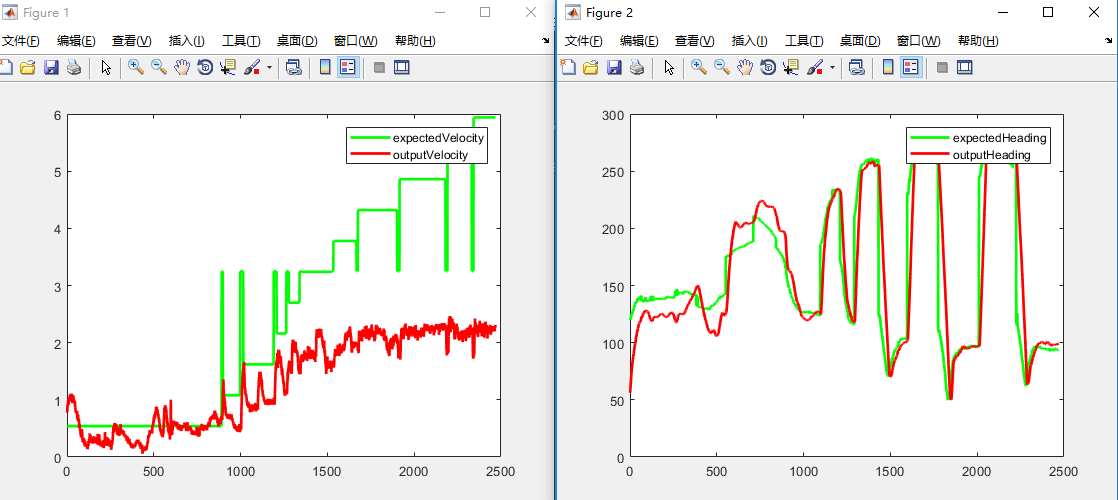• 利用MATLAB数据进行切片并绘制图表 文章目录利用MATLAB数据进行切片并绘制图表1 读取txt文件2 对矩阵进行切片，提取信息3 使用MATLAB绘图4 附录——完整代码 1 读取txt文件 使用MATLAB中的load函数加载记事本...
利用MATLAB对数据进行切片并绘制图表

文章目录
利用MATLAB对数据进行切片并绘制图表1 读取txt文件2 对矩阵进行切片，提取信息3 使用MATLAB绘图4 附录——完整代码

1 读取txt文件
filename = '2019-03-24 10_04_47-iip-header.txt';
[Rows, Columuns] = size(data);		%如果是仅仅获取行数，则使用size(data,1)

在读取的时候，load函数出现了错误，原因是load函数只能读取完全为数字的文本，而打开这个文件发现最后一行数据稍微有点缺失：因此改为importdata函数
data = importdata(filename);

2 对矩阵进行切片，提取信息
把需要的数据读取成列向量
根据接口协议，把需要的数据进行切片，得到列向量
longi = data( : , 31);
lati = data( : , 32);
expectedVelocity = data( : ,96);
outputVelocity = data( : ,43);

3 使用MATLAB绘图
Matlab中使用figure和plot函数绘图，期望值用绿色表示，实际值用红色表示
figure
plot(expectedVelocity,'g','linewidth',2);
hold on
plot(outputVelocity,'r','linewidth',2);
legend('expectedVelocity','outputVelocity');
figure
hold on

得到图形4 附录——完整代码
clc;
clear all;
close all;
data = importdata(filename);
longi = data( : , 31);
lati = data( : , 32);
expectedVelocity = data( : ,96);
outputVelocity = data( : ,43);
figure
plot(expectedVelocity,'g','linewidth',2);
hold on
plot(outputVelocity,'r','linewidth',2);
legend('expectedVelocity','outputVelocity');
figure
hold on


展开全文• ## matlab切片操作

千次阅读 2021-04-02 16:31:06
matlab切片访问 matlab切片访问包括索引值所在的行列，且索引值从1开始，c或者python是从0开始 >> ab = [1,2,1,3; 2,6,5,7; 7,3,2,0] ab = 1 2 1 3 2 6 5 7 7 3 2 0 >> size(ab) ans = 3 4 ...
matlab切片访问
matlab切片访问包括索引值所在的行列，且索引值从1开始，c或者python是从0开始
>> ab = [1,2,1,3; 2,6,5,7; 7,3,2,0]

ab =

1     2     1     3
2     6     5     7
7     3     2     0

>> size(ab)
ans =

3     4

>> b = ab(:, 2:4) %取第2-4列所在所有行，包括第2和第4

b =
2     1     3
6     5     7
3     2     0

>> b = ab(:, 3:4)
b =

1     3
5     7
2     0
>> b(:,2:3)=0 % 给第2和第3列所在所有行的元素赋值0
b =

1     0     0     3
2     0     0     7
7     0     0     0

%%沿x或y轴翻转 类似cv2.filp()操作
A = [ 1 2 3; 4 5 6; 7 8 9];
B = A(:,end:-1:1) %沿垂直方向Y轴翻转
% B = A(end:-1:1, :) %沿水平方向X轴翻转
% 前后左右翻转 A_flip = A(end : -1 : 1, end : -1 : 1)
C = circshift(B,[0,1]) %翻转之后再进行列移位，右移1位

B =
3     2     1
6     5     4
9     8     7

C =
1     3     2
4     6     5
7     9     8

展开全文• 利用MATLAB 7.0的"slice"切片功能将三维表示的数据，通过对图形的线型、立面、色彩、渲染、光线、视角等的控制，可形象表现数据四维特性。
• 1 2 3 4 5 ... %% ======切片图和切片等位线图======= ... %利用 slice 和 contourslice 表现 MATLAB 提供的无限大水体中水下射流速度数据 flow 。 flow 是一组定义在三维空间上的函数数据。 ...
 1 2 3 4 5 6 7 8 9 10 11 12 13 14 15 16 17 18 19 20  %% ======切片图和切片等位线图======= %利用 slice 和 contourslice 表现 MATLAB 提供的无限大水体中水下射流速度数据 flow 。 flow 是一组定义在三维空间上的函数数据。 %在本例中，从图中的色标尺可知，深红色表示“正速度”（向图的左方），深蓝表示“负速度”（向图的右方）。 % 以下指令用切面上的色彩表现射流速度 [X,Y,Z,V]=flow; % 取 4 个 的射流数据矩阵， V 是射流速度。 x1=min(min(min(X)));x2=max(max(max(X))); % 取 x 坐标上下限 y1=min(min(min(Y)));y2=max(max(max(Y))); % 取 y 坐标上下限 z1=min(min(min(Z)));z2=max(max(max(Z))); % 取 z 坐标上下限 sx=linspace(x1+1.2,x2,5); % 确定 5 个垂直 x 轴的切面坐标 sy=0; % 在 y=0 处，取垂直 y 轴的切面 sz=0; % 在 z=0 处，取垂直 z 轴的切面 figure; slice(X,Y,Z,V,sx,sy,sz); % 画切片图 view([-12,30]);shading interp;colormap jet;axis off;colorbar; % 以下指令用等位线表现射流速度 v1=min(min(min(V)));v2=max(max(max(V))); % 射流速度上下限 cv=linspace(v1,v2,15); % 在射流上下限之间取 15 条等位线 figure; contourslice(X,Y,Z,V,sx,sy,sz,cv);view([-12,30]); colormap jet;colorbar;box on;

下面两段程序均不便上图，自己拿到Matlab里面运行一下看效果吧。
1 2 3 4 5 6 7 8 9 10 11 12 13 14 15 16 17 18 19 20 21 22 23 24 25  %% =======动态图形========= %简单二维示例-彗星状轨迹图 figure; n=10;t=n*pi*(0:0.0005:1);x=sin(t);y=cos(t); plot(x,y,'g');axis square;hold on comet(x,y,0.01);hold off %卫星返回地球的运动轨线示意 figure; R0=1; % 以地球半径为一个单位 a=12*R0;b=9*R0;T0=2*pi; %T0 是轨道周期 T=5*T0;dt=pi/100;t=[0:dt:T]'; f=sqrt(a^2-b^2); % 地球与另一焦点的距离 th=12.5*pi/180; % 卫星轨道与 x-y 平面的倾角 E=exp(-t/20); % 轨道收缩率 x=E.*(a*cos(t)-f);y=E.*(b*cos(th)*sin(t));z=E.*(b*sin(th)*sin(t)); plot3(x,y,z,'g') % 画全程轨线 [X,Y,Z]=sphere(30);X=R0*X;Y=R0*Y;Z=R0*Z; % 获得单位球坐标 grid on,hold on,surf(X,Y,Z),shading interp % 画地球 x1=-18*R0;x2=6*R0;y1=-12*R0;y2=12*R0;z1=-6*R0;z2=6*R0; axis([x1 x2 y1 y2 z1 z2]) % 确定坐标范围 view([117 37]),comet3(x,y,z,0.02),hold off % 设视角、画运动轨线 %色彩变幻‘在 256 色情况下,才可被正确执行.图片刷新可能会卡,单独执行spinmap可查看到效果 figure; peaks; spinmap;
1 2 3 4 5 6 7 8 9 10 11 12  %% =======影片动画 ======= %三维图形的影片动画 figure; shg,x=3*pi*(-1:0.05:1);y=x;[X,Y]=meshgrid(x,y); R=sqrt(X.^2+Y.^2)+eps; Z=sin(R)./R; h=surf(X,Y,Z);colormap(cool);axis off n=12;mmm=moviein(n); %预设画面矩阵。新版完全可以取消此指令 。 for i=1:n rotate(h,[0 0 1],25); %是图形绕 z 轴旋转 25 度 / 每次 mmm(:,i)=getframe; %捕获画面。新版改为 mmm(i)=getframe 。 end movie(mmm,5,10) %以每秒10帧速度，重复播放5次 
展开全文• % 指定切片的位置在xyz轴 h = slice(x,y,z,v,[-1 -.75 -.5],[],); set(h,'EdgeColor','none',... 'FaceColor','interp',... 'FaceAlpha','interp') alpha('color') alphamap('rampdown') alphamap('increase',.1...
例子1
slice用法介绍
load mri
D = double(squeeze(D));
D(D==0)=nan;
h = slice(D, [], [], 1:size(D,3));
set(h, 'EdgeColor','none', 'FaceColor','interp')
alpha(.4)例子2
官方文档介绍
[x,y,z] = meshgrid(-1.25:.1:-.25,-2:.2:2,-2:.1:2);
v = x.*exp(-x.^2-y.^2-z.^2);
% 指定切片的位置在xyz轴
h = slice(x,y,z,v,[-1 -.75 -.5],[],);
set(h,'EdgeColor','none',...
'FaceColor','interp',...
'FaceAlpha','interp')
alpha('color')

alphamap('rampdown')
alphamap('increase',.1)
colormap hsvpcolor3效果
%% example1
[x,y,z] = meshgrid(-2:.05:2,-2:.1:2,-2:.1:2);
v = x.*exp(-x.^2-y.^2-z.^2) + 1;
figure
pcolor3(v)
axis tight%% example2
figure
pcolor3(x,y,z,v,'edgealpha',.1)
rgbmap('blue','white','red')
colorbar
caxis([0.8 1.2])
% camlight
view(160,36)%% example3
figure
pcolor3(x,y,z,v,'cubic','edgealpha',.1)
% pcolor3(x,y,z,v,'alphalim',[1 1.2],'cubic','edgealpha',.1)
rgbmap('blue','white','red')
colorbar
caxis([0.8 1.2])
% camlight
view(160,36)%% example4
[lon,lat,z] = meshgrid(-180:3:180,-80:2:80,0:100:2000);
T = 30 - .1*abs(lat) - .01*z + sind(lon);
figure
pcolor3(lon,lat,z,T,'direct')
% pcolor3(lon,lat,z,T,'direct','alphalim','auto')
% format:
xlabel('longitude')
ylabel('latitude')
zlabel('elevation (m)')
axis tight
set(gca,'xtick',[-180 -90 0 90 180],...
'ytick',[-45 0 45])
plot(c.long,c.lat)旋转绘图
方法1 spinningGIF
load mri
D = double(squeeze(D));
D(D==0)=nan;
h = slice(D, [], [], 1:size(D,3));
set(h, 'EdgeColor','none', 'FaceColor','interp')
alpha(.4)

spinningGIF('123')

% spinningGIF(fname): makes a spinning GIF of the current plot and saves it
% Usage: make your 3D plot (using plot3(...) or scatter3(...) etc.) and
% then call SpinningGIF with the file name that you want
function spinningGIF(fname)
axis off
%     view(0,10)
center = get(gca, 'CameraTarget');
pos = get(gca, 'CameraPosition');
angles = 0:0.02*pi:2*pi;
for ii=1:length(angles)
angle = angles(ii);
set(gca, 'CameraPosition', [center(1) + radius * cos(angle),...
pos(3)]);
drawnow;
frame = getframe(1);
im = frame2im(frame);
[imind,cm] = rgb2ind(im,256);
if ii == 1
imwrite(imind,cm,fname,'gif', 'Loopcount',inf);
else
imwrite(imind,cm,fname,'gif','WriteMode','append','DelayTime', 0.25);
end
end
end

方法2
OptionZ.FrameRate=15;OptionZ.Duration=5.5;OptionZ.Periodic=true;

方法3
load mri
D = double(squeeze(D));
D(D==0)=nan;
h = slice(D, [], [], 1:size(D,3));
set(h, 'EdgeColor','none', 'FaceColor','interp')
alpha(.4)

for az=0:5:355
view(az,36)
drawnow;
end

展开全文• zigzag代码matlab STL-切片机 这是一个基本的 MATLAB 代码，它以 STL 文件作为输入并提供切片平面和模型的交集。 STL文件的基础知识 STL 文件中的数据以三角形曲面网格（也称为三角形镶嵌）的形式存储。 这使得 3D ...
• 此函数的输出是新的 MATLAB 图窗、轴句柄向量和切片的 y 坐标。 句法： 图像切片(z) imageslices（x，y，z） 图像切片（z，Nslices） 图像切片（z，Nslices，ylimits） imageslices（z，ylimits） 图像切片（___，...
• 用于多通道图像（例如，高光谱、MRI、卫星或任何其他类型的具有超过 1 个波段的图像数据）的图像立方体切片器的实现。 图像立方体切片器在左侧面板中包含一个图像带屏幕，其中显示了图像平面（使用 imshow() 或 ...
• 这个简单的功能允许用户查看特定 .nii 格式 MRI 扫描的矢状、冠状和水平切片。 您需要做的就是输入某个点的 x、y 和 z 坐标，以便能够查看通过该点的切片。 颜色图自动设置为灰色，但可以根据需要进行更改。 该函数...
• MATLAB二维数据可视化 MATLAB三维数据可视化 MATLAB四维数据可视化 视频教程入口 第一章：二维数据可视化 01. 线形图-plot函数 13:05 02. 线形图-简单线形图和复合线形图 7:26 03. 线形图-属性及属性的...
• 使用箭头键更改切片湾'1','2','3'键改变视图C。 鼠标右键单击选项菜单d. 鼠标左键单击/按住以调整窗口级别一世。 左右改变窗口中心ii. 上下更改窗口宽度e. '+' 用于放大，图像将在最后一次单击鼠标时重新居中。 ...
• imagesc3D 使用来自 3 个垂直方向的 imagesc 函数显示 3D 图像视图（即 3（e1-e2 平面）、1（e2-e3 平面）、2（e3-e1 平面）、）） 通过基于鼠标的切片浏览和窗口进行逐片时尚电平调节控制。 用法： imagesc3D(...) ...
• spacetimevideoslice 允许您创建视频数据的直观二维可视化。 这对于突出显示时间变化特别有用，例如运动和颜色变化。 切片可以水平（图像高度上恒定位置的时间条）或垂直（沿图像宽度的恒定位置的条）制作，并沿...
• 学习血管分割和三维重建的时候，知网上检索到很多血管切片的三维重建，就想着找一些资料，着手复现一下。...Matlab代码实现 [数学建模] 1. 血管的三维重建 下载数据集后，把数据和源码保存到一个文件夹下边
• 此功能旨在查看体积数据的体积切片图像。 您可以使用交互式窗口/级别功能调整图像的对比度和动态范围。 为方便起见，提供了对数压缩直方图面积图。 此应用程序中使用的交互式滑块可从以下 URL 获得...
• 体积浏览器通过静态和动画切片（后者可以保存为电影）、轮廓切片和等值面显示标量 3-D 数据集，主要是三个变量的函数。 此版本与 V3D 之间的主要区别是： 大多数菜单按钮已被 Matlab 标准菜单栏中的按钮所取代。 ...
• 与其绘制 3D 体积，不如绘制一系列切片。 与 surf() 类似，但在轴之一中没有插值。... 请注意，由于切片的宽度是有限的（宽度是轴点的间距），因此，如果手动设置轴限制，则可能会截断数据的末端。
• 此 GUI 设计用于查看 ... 用户可以翻阅图像的各个切片，也可以查看所选切片的水平和垂直剖面。 数据应该是维度为 y、x、z 的 3D 矩阵。 您可以使用置换按钮更改数据的方向，并在查看数据的幅度、实部和虚部之间切换。
• 利用MATLAB 7.0的"slice"切片功能将三维表示的数据，通过对图形的线型、立面、色彩、渲染、光线、视角等的控制，可形象表现数据四维特性。信息可视化
• 基于MATLAB的人体切片图像处理与分析.pdf
• 为提高STL模型的切片效率,提出一种基于MATLAB的STL模型切片分层新算法。首先对STL模型进行数据预处理,筛选出只与切平面相交的三角片集合,然后利用相邻三角面片边的拓扑关系依次求交,获得各层截面轮廓信息。实验结果...
• 这个小型 GUI 可以查看 DM3 格式（数码显微照片）的 3D 数据堆栈中的不同切片
• 建议使用其他功能生成切片轮廓数据。 （例如Sunil Bhandari创建的函数slice_stl_create_pa th（triangles，slice_height）） [xy_points] = add_features（轮廓_xy_points，shell_layers，shell_thickness，base_...
• 这是用于各种神经成像预处理操作（注册，切片，去噪，分割等）的MATLAB代码，其最初旨在处理常规临床数据（因此而得名）。 它以nifti文件（如.nii或.nii.gz）作为输入，并生成此数据的副本，并对其应用了所需的...
• 基于MATLAB实现地震速度切片成图 (1).pdf
• 这是用于各种神经成像预处理操作（注册，切片，去噪，分割等）的MATLAB代码，其最初旨在处理常规临床数据（因此得名）。 它以nifti文件（如.nii或.nii.gz）作为输入，并生成此数据的副本，并对其应用了所需的...
• 之前写了医学影像三维重建的文章，许多小伙伴都来问测试数据的model是怎么生成的。这个其实是MATLAB基础，但由于询问的人太多，就在这里统一回复了。内容比较简单，适用于刚开始用MATLAB的伙伴。三维数组 三维重建
• matlab开发-高光谱图像立体切片机。图像切片器，用于可视化多通道图像数据
• matlab图片叠加的代码切片显示 概述 Slice Display是用于可视化fMRI数据MATLAB工具箱。 除了标准的fMRI显示器外，它还可以制作双编码图像（同时显示对比度估计值和无阈值t统计图）。 数据可视化可以完全编写脚本，......

# matlab数据切片matlab 订阅